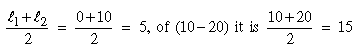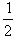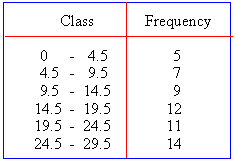Support the Monkey! Tell All your Friends and TeachersHome MonkeyNotes Printable Notes Digital Library Study Guides Study Smart Parents Tips College Planning Test Prep Fun Zone Help / FAQ How to Cite Request a New Title

According to the class-intervals in classification the following terms are used :

i) Class-limits : A class is formed within the two values. These values are known as the class-limits of that class. The lower value is called the lower limit and is denoted by l1 while the higher value is called the upper limit of the class and is denoted by l2. In the example given above, the first class-interval has l1 = 0 and l2 = 10.

ii) Magnitude of the class-intervals : The difference between the upper and lower limits of a class is called the magnitude or length or width of a class and is denoted by ' i ' or ' c '. Thus i º ( l2 - l1).

iii) Mid-value or class-mark : The arithmetical average of the two class limits (i.e. the lower limit and the upper limit ) is called the mid-value or the class mark of that class-interval. For example, the mid-value of the class-interval ( 0 - 10 ) isand so on.

iv) Class frequency : The units of the data belong to any one of the groups or classes. The total number of these units is known as the frequency of that class and is denoted by fi or simply f. In the above example, the frequencies of the classes in the given order are 5, 9, 32, 34 and 40 respectively.

Classification is of two types according to the class-intervals - (i) Exclusive Method (ii) Inclusive Method.

i) Exclusive Method : In this method the upper limit of a class becomes the lower limit of the next class. It is called ' Exclusive ' as we do not put any item that is equal to the upper limit of a class in the same class; we put it in the next class, i.e. the upper limits of classes are excluded from them. For example, a person of age 20 years will not be included in the class-interval ( 10 - 20 ) but taken in the next class ( 20 - 30 ), since in the class interval ( 10 - 20 ) only units ranging from 10 - 19 are included. The exclusive-types of class-intervals can also be expressed as :

0 and below 10    or    0 - 9.9
10 and below 20     or    10 - 19.9
20 and below 30    or    20 - 29.9 and so on.

ii) Inclusive Method : In this method the upper limit of any class interval is kept in the same class-interval. In this method the upper limit of a previous class is less by 1 from the lower limit of the next class interval. In short this method allows a class-interval to include both its lower and upper limits within it. For example :

Table - 2Your browser does not support the IFRAME tag.

Class boundaries : Weights are recorded to the nearest Kg The class-intervals 60 - 62 includes all measurements from 59.50000... to 62.50000 ... Kg ; the variable being a continuous one. These numbers, indicated briefly by the exact numbers 59.5 and 62.5, are called class-boundaries or true class limits. The smaller number 59.5 is the lower class boundary and the larger one 62.5 is the upper class boundary.

In any problem if the class-intervals are given as the inclusive type, then they should first be converted into the exclusive-type . For this we require a correction factor.

Correction factor =( the upper limit of a class - the lower limit of the next class) which is generally 0.5.

Now you subtract it from the lower limits and add it to the upper limits of the class-intervals given in the inclusive-method. The class-intervals given above can be written after correction as :To obtain class-intervals when their mid-values are given, use the following formulae :

Lower limit (l1 ) = m - i/2 and upper limit (l2 ) = m + i/2

where m = mid-value and i = class-length.

Index

2.1 Introduction
2.2 Tabulation
2.3 Classification
2.4 Methods of classification
2.5 Relative frequency distribution
2.6 Cumulative frequency
2.7 Bivariate frequency distribution

Chapter 3Search: All Products Books Popular Music Classical Music Video DVD Toys & Games Electronics Software Tools & Hardware Outdoor Living Kitchen & Housewares Camera & Photo Cell Phones Keywords: